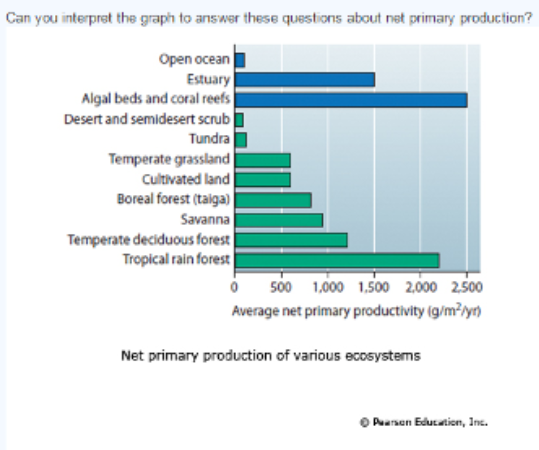# Problem: What do the bars on this graph represent?A) The amount of solar energy converted to chemical energy in organic compounds for a given area per year.B) The average amount of solar energy absorbed by various ecosystems. C) The percentage of Earth's total primary productivity contributed by various ecosystems.D) The solar energy available to each ecosystem as determined by its geographic location.

###### FREE Expert Solution

In an ecosystem:

Energy flow: unidirectional

- energy is transferred from producers to consumers with a loss in energy (as heat) for each step###### Problem Details

What do the bars on this graph represent?A) The amount of solar energy converted to chemical energy in organic compounds for a given area per year.

B) The average amount of solar energy absorbed by various ecosystems.

C) The percentage of Earth's total primary productivity contributed by various ecosystems.

D) The solar energy available to each ecosystem as determined by its geographic location.